## Isomer Enumeration of Simple Hydrocarbons

Ma Chengyuan, Li Qixiang,Abstract

Can we count hydrocarbon isomers systematically and accurately? This article demonstrates a neat solution by introducing generating functions and Pólya's Counting Theorem based on high-school-level mathematics and chemistry knowledge. Starting with alkyls, we derive formulae to enumerate acyclic isomers (stereoisomerism not considered) of alkanes, monoalkenes, monoalkynes, benzene derivatives, disubstituted alkanes and dienes. We then check our results against manual enumeration to show their correctness. We also explore the asymptotic behavior of isomerism and empirically propose approximation formulae for counting isomers of the aforementioned hydrocarbons. Compared to results from literature, we strive to reduce advanced mathematical concepts involved to eliminate barriers for inquisitive high school readers, who may gain a more in-depth understanding of hydrocarbon structure and train their computational thinking. Compared to results from Chinese literature, our derivation is more concise, flexible and extendable.

Keywords： Hydrocarbon ; Isomer ; Enumeration ; Pólya's Counting Theorem

Ma Chengyuan. Isomer Enumeration of Simple Hydrocarbons. University Chemistry[J], 2022, 37(1): 2103067-0 doi:10.3866/PKU.DXHX202103067

●    所有碳成四键、氢成一键的结构式对应的有机物都现实存在。

●    碳链相同的两有机物结构相同，即只考虑碳链异构，不考虑立体异构。

●    如不特殊说明，则所有本文涉及到的所有烃无环(苯环除外)。

### 1.1 生成函数

1) 去掉2个原子，用剩下的原子组成结构A的方案数{0, 0, a0, a1, a2, …}对应的生成函数是x2A(x)。

2) 组成两个相同的结构A的方案数{a0, 0, a1, 0, a2, 0…}对应的生成函数是A(x2)。

3) 要么组成结构A，要么组成结构B的方案数{an + bn}对应的生成函数是A(x) + B(x)。

4) 用一些原子组成结构A，另一些原子组成结构B的方案数{∑i+j=naibj}对应的生成函数就是A(x)B(x)。

### 1.2 Pólya计数定理

${s_\pi }\left\{ {f(x)} \right\} = \prod\limits_i {{f^{{c_i}}}} \left( {{x^i}} \right)$

${\mathcal{S}_k}\left\{ {f(x)} \right\} = \frac{1}{{k!}}\sum\limits_{\pi \in {S_k}} {{s_\pi }} \left\{ {f(x)} \right\}$

$\begin{array}{l} {\mathcal{S}_2}\left\{ {f(x)} \right\} = \frac{1}{2}\left[ {{f^2}(x) + f\left( {{x^2}} \right)} \right] \\ {\mathcal{S}_3}\left\{ {f(x)} \right\} = \frac{1}{6}\left[ {{f^3}(x) + 3f(x)f\left( {{x^2}} \right) + 2f\left( {{x^3}} \right)} \right] \\ {\mathcal{S}_4}\left\{ {f(x)} \right\} = \frac{1}{{24}}\left[ {{f^4}(x) + 6{f^2}(x)f\left( {{x^2}} \right) + 3{f^2}\left( {{x^2}} \right) + 8f(x)f\left( {{x^3}} \right) + 6f\left( {{x^4}} \right)} \right] \\ \end{array}$

### 2.1 烷基的同分异构体计数

1) 烷基中所有的碳都成四根单键。

2) 烷基当中成半键的碳原子有特殊性(下文不妨称其为“根碳原子”)，使其可以成为计数的基准点。

### 图1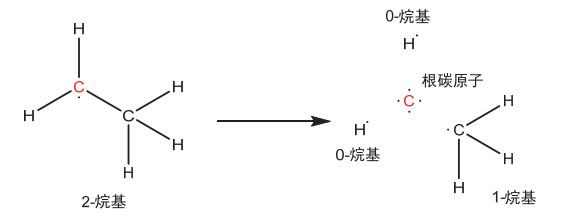(4)

$\begin{array}{l}\;\;{T_0}(x) = 1 \\ {T_{h + 1}}(x) = 1 + x{S_3}\left\{ {{T_h}(x)} \right\} \\ \;\;\;\;\;\;\;\;\;\;\;\;= 1 + \frac{x}{6}\left[ {T_h^3(x) + 3{T_h}(x){T_h}\left( {{x^2}} \right) + 2{T_h}\left( {{x^3}} \right)} \right] \\ \end{array}$

$\begin{array}{l} {T_0}(x) = 1 \\ {T_1}(x) = 1 + x \\ {T_2}(x) = 1 + x + {x^2} + \cdots \\ \vdots \\ {T_7}(x) = 1 + x + {x^2} + 2{x^3} + 4{x^4} + 8{x^5} + 17{x^6} + 39{x^7} + \cdots \\ {T_8}(x) = 1 + x + {x^2} + 2{x^3} + 4{x^4} + 8{x^5} + 17{x^6} + 39{x^7} + 89{x^8} + \cdots \\ \end{array}$

$\begin{array}{l}\;\;\;{t_{h, 0}} = 1 \\ \;\;\;{t_{0, n}} = 0 \\ {t_{h + 1, n + 1}} = \frac{1}{6}\left( {\sum\limits_{i + j + k = n} {{t_{h, i}}} {t_{h, j}}{t_{h, k}} + 3\sum\limits_{i + 2j = n} {{t_{h, i}}} {t_{h, j}} + 2\sum\limits_{3i = n} {{t_{h, i}}} } \right) \\ \;(h, {\text{ }}n = 0, {\text{ }}1, {\text{ }}2, {\text{ }}3{\text{ }}, {\text{ }} \cdots ) \\ \end{array}$

### 图2### 图3${C_{2h}}(x) = {\mathcal{S}_2}\left\{ {{T_h}(x) - {T_{h - 1}}(x)} \right\}$

### 图4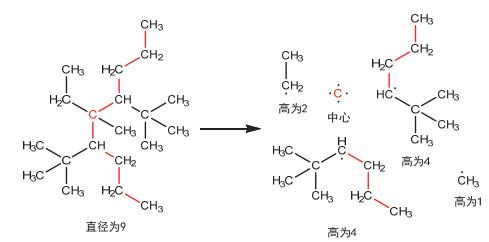${C_{2h + 1}}(x) = x\left[ {{\mathcal{S}_4}\left\{ {{T_h}(x)} \right\} - {\mathcal{S}_4}\left\{ {{T_{h - 1}}(x)} \right\} - \left( {{T_h}(x) - {T_{h - 1}}(x)} \right){\mathcal{S}_3}\left\{ {{T_{h - 1}}(x)} \right\}} \right]$

$\begin{array}{l} C(x) = \sum\limits_{d = 1}^\infty {{C_d}} (x) \\ \;\;\;\;\;\;\;\; = x + {x^2} + {x^3} + 2{x^4} + 3{x^5} + 5{x^6} + 9{x^7} + 18{x^8} + 35{x^9} + 75{x^{10}} + 159{x^{11}} + 355{x^{12}} + 802{x^{13}} + \cdots \\ \end{array}$

### 图5$\begin{array}{l} {C_{{\text{alkene}}}}(x) = {x^2}{\mathcal{S}_2}\left\{ {{\mathcal{S}_2}\left\{ {T(x)} \right\}} \right\} \\ \;\;\;\;\;\;\;\;\;\;\;\; = {x^2} + {x^3} + 3{x^4} + 5{x^5} + 13{x^6} + 27{x^7} + 66{x^8} \cdots \\ \end{array}$

$\left( {\begin{array}{*{20}{c}} {{\text{X}} \to {\text{X}}} \\ {{\text{Y}} \to {\text{Y}}} \\ {{\text{Z}} \to {\text{Z}}} \\ {{\text{W}} \to {\text{W}}} \end{array}} \right), \left( {\begin{array}{*{20}{c}} {{\text{X}} \to {\text{Z}}} \\ {{\text{Y}} \to {\text{W}}} \\ {{\text{Z}} \to {\text{X}}} \\ {{\text{W}} \to {\text{Y}}} \end{array}} \right), \left( {\begin{array}{*{20}{c}} {{\text{X}} \to {\text{Y}}} \\ {{\text{Y}} \to {\text{X}}} \\ {{\text{Z}} \to {\text{W}}} \\ {{\text{W}} \to {\text{Z}}} \end{array}} \right), \left( {\begin{array}{*{20}{c}} {{\text{X}} \to {\text{W}}} \\ {{\text{Y}} \to {\text{Z}}} \\ {{\text{Z}} \to {\text{Y}}} \\ {{\text{W}} \to {\text{X}}} \end{array}} \right)$

$\begin{array}{l} {{C'}_{{\text{alkene}}}}(x) = \frac{{{x^2}}}{4}\left[ {{T^4}(x) + 3{T^2}({x^2})} \right] \\ \;\;\;\;\; \;\;\;\;\;\;\;\;\;\;= {x^2} + {x^3} + 4{x^4} + 6{x^5} + 17{x^6} + 36{x^7} + 92{x^8} \cdots \\ \end{array}$

#### 2.4 一炔烃的同分异构体计数

$\begin{array}{l} {C_{{\text{alkyne}}}}(x) = {x^2}{\mathcal{S}_2}\left\{ {T(x)} \right\} \\ \;\;\;\;\;\;\;\;\;\;\;\;\;\;\;= {x^2} + {x^3} + 2{x^4} + 3{x^5} + 7{x^6} + 14{x^7} + 32{x^8} \cdots \\ \end{array}$

#### 2.5 苯的衍生物的同分异构体计数

$\begin{array}{l} {C_{{\text{benzene}}}}(x) = \frac{{{x^6}}}{{12}}\left[ {{T^6}(x) + 3{T^2}(x){T^2}\left( {{x^2}} \right) + 4{T^3}\left( {{x^2}} \right) + 2{T^2}\left( {{x^3}} \right) + 2T\left( {{x^6}} \right)} \right] \\ \;\;\;\;\;\;\;\;\;\;\;\;\;\;\;\;= {x^6} + {x^7} + 4{x^8} + 22{x^9} + 51{x^{10}} + 136{x^{11}} + \cdots \\ \end{array}$

##### 2.6.1 异官能团

${B_h}(x) = {\left( {x{\mathcal{S}_2}\left\{ {T(x)} \right\}} \right)^h}$

$\begin{array}{l} B(x) = \sum\limits_{h = 0}^\infty {{{\left( {x{\mathcal{S}_2}\left\{ {T(x)} \right\}} \right)}^h}} \\ \;\;\;\;\;\;\; = x + 2{x^2} + 5{x^3} + 12{x^4} + 31{x^5} + 80{x^6} + 210{x^7} \cdots \\ \end{array}$

##### 2.6.2 同官能团

${B'_{2h}}(x) = {\mathcal{S}_2}\left\{ {{{\left( {x{\mathcal{S}_2}\left\{ {T(x)} \right\}} \right)}^h}} \right\}$

${B'_{2h + 1}}(x) = \left( {x{\mathcal{S}_2}\left\{ {T(x)} \right\}} \right){\mathcal{S}_2}\left\{ {{{\left( {x{\mathcal{S}_2}\left\{ {T(x)} \right\}} \right)}^h}} \right\}$

$\begin{array}{l} B'(x) = \sum\limits_{h = 0}^\infty {{{B'}_{2h}}} (x) + \sum\limits_{h = 0}^\infty {{{B'}_{2h + 1}}} (x) \\ \;\;\;\;\;\;\; = x + 2{x^2} + 4{x^3} + 9{x^4} + 21{x^5} + 52{x^6} + 129{x^7} \cdots \\ \end{array}$

### 图6$\begin{array}{l} \;\;{A_{2h}}(x) = {\mathcal{S}_2}\left\{ {{x^2}T(x){\mathcal{S}_2}\left\{ {T(x)} \right\}{{\left( {x{\mathcal{S}_2}\left\{ {T(x)} \right\}} \right)}^h}} \right\} \\ {B_{{\text{alkene}}}}(x) = \sum\limits_{h = 0}^\infty {{A_{2h}}} (x) + \sum\limits_{h = 0}^\infty x {\mathcal{S}_2}\left\{ {T(x)} \right\}{A_{2h}}(x) \\ \;\;\;\;\;\;\;\;\;\;\;\;\;= 1{x^4} + 3{x^5} + 11{x^6} + 31{x^7} + 93{x^8} + 262{x^9} + 748{x^{10}} \cdots \\ \end{array}$

## 3 渐进性质探究

### 图7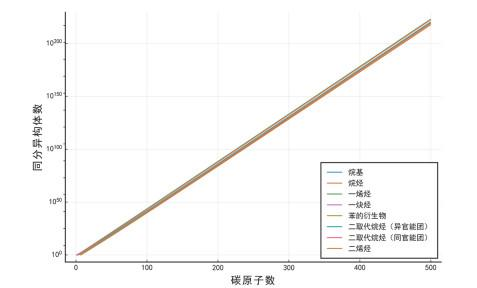1) 烃类同分异构体数目的变化由指数增长的主导，与我们的直觉相符；

2) 同时，指数增长的底数不随化合物的种类有明显变化。看似复杂的烃类并不比简单的烷基同分异构体数目增长快(单从指数增长的角度)，这是与我们直觉是相悖的。

### 图8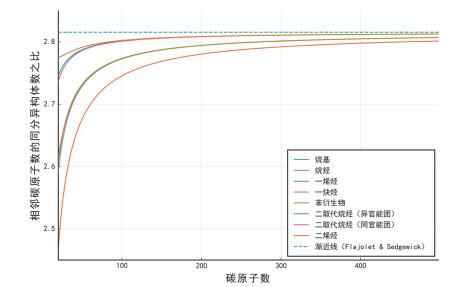### 图9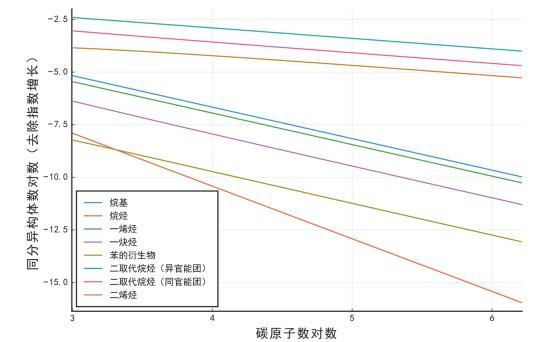化合物类型 k C 烷基 −1.498 0.512 烷烃 −2.502 0.664 一烯烃 −1.498 0.386 一炔烃 −1.519 0.155 苯的衍生物 −1.508 0.025 二取代烷烃(异官能团) −0.498 0.404 二取代烷烃(同官能团) −0.509 0.215 二烯烃 −0.468 0.095

 化合物类型 k C 烷基 −3/2 0.517 烷烃 −5/2 0.657 一烯烃 −3/2 0.390 一炔烃 −3/2 0.140 苯的衍生物 −3/2 0.024 二取代烷烃(异官能团) −1/2 0.408 二取代烷烃(同官能团) −1/2 0.206 二烯烃 −1/2 0.113

### 图10## 参考文献 原文顺序 文献年度倒序 文中引用次数倒序 被引期刊影响因子

Cayley E. Chem. Ber. 1875, 8 (2), 10569.

Pólya G. Acta Math. 1937, 68 (1), 145.

Pólya G. ; Read R. C. Combinatorial Enumeration of Groups, Graphs, and Chemical Compounds Berlin, Germany: Springer-Verlag, 2012.

Rains, E. M.; Sloane, N. J. A. J. Integer Seq. 1999, 2, Art 99.1.1.

Hermann F. Chem. Ber. 1880, 13 (1), 792.

Henze H. R. ; Blaire C. M. J. Am. Chem. Soc. 1931, 53 (8), 3077.

Read, R. C. The Enumeration of Acyclic Chemical Compounds. In Chemical Applications of Graph Theory; Babalan, A. T. Ed.; Academic Press: New York, USA, 1976; pp. 26-61.

Robinson R. W. ; HarryF ; Babalan A. T. Tetrahedron 1976, 32 (3), 355.

Flajolet P. ; Sedgewick R. Analytic Combinatorics Cambridge, UK: Cambridge University Press, 2009, pp. 456- 457.

/

 〈〉